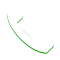0

# I need to run a 1 HP 750 Watt water pump and a 12 V inverter battery at the same time. Can a 2 KW generator handle it?

By 12 volt inverter I assume you are talking about a normal 500 VA inverter. Power consumption during full load charging = 12*15 => 180 watts Load other than inverter let’s say 1 tv (80 watt), fan(80 watt) and light (20 watt) =80+80+20=>180 watt 1 H.P motor = 746 Watt Normal running current = 746/220 Starting current = 5 times =>5*746/220 Starting power = 220*starting current => 220*5*746/220=>5*746=3730 watt Total starting load = 3730 + 180 + 180 => 4090 Watt Normal load = 746 + 180+180+746 = 1100 Watt I would recommend going for 5 KW. You will be having issues later if starting 1 hp induction motor on 2 KW generator.For COD  Orders Call Us